# spectralcluster

Spectral clustering

## Syntax

``idx = spectralcluster(X,k)``
``idx = spectralcluster(S,k,'Distance','precomputed')``
``idx = spectralcluster(___,Name,Value)``
``[idx,V] = spectralcluster(___)``
``[idx,V,D] = spectralcluster(___)``

## Description

example

````idx = spectralcluster(X,k)` partitions observations in the n-by-p data matrix `X` into `k` clusters using the spectral clustering algorithm (see Algorithms). `spectralcluster` returns an n-by-1 vector `idx` containing cluster indices of each observation.```

example

````idx = spectralcluster(S,k,'Distance','precomputed')` returns a vector of cluster indices for `S`, the similarity matrix (or adjacency matrix) of a similarity graph. `S` can be the output of `adjacency`.To use a similarity matrix as the first input, you must specify `'Distance','precomputed'`.```

example

````idx = spectralcluster(___,Name,Value)` specifies additional options using one or more name-value pair arguments in addition to the input arguments in previous syntaxes. For example, you can specify `'SimilarityGraph','epsilon'` to construct a similarity graph using the radius search method.```
````[idx,V] = spectralcluster(___)` also returns the eigenvectors `V` corresponding to the `k` smallest eigenvalues of the Laplacian matrix.```

example

````[idx,V,D] = spectralcluster(___)` also returns a vector `D` containing the `k` smallest eigenvalues of the Laplacian matrix.```

## Examples

collapse all

Cluster a 2-D circular data set using spectral clustering with the default Euclidean distance metric.

Generate synthetic data that contains two noisy circles.

```rng('default') % For reproducibility % Parameters for data generation N = 300; % Size of each cluster r1 = 2; % Radius of first circle r2 = 4; % Radius of second circle theta = linspace(0,2*pi,N)'; X1 = r1*[cos(theta),sin(theta)]+ rand(N,1); X2 = r2*[cos(theta),sin(theta)]+ rand(N,1); X = [X1;X2]; % Noisy 2-D circular data set```

Find two clusters in the data by using spectral clustering.

`idx = spectralcluster(X,2);`

Visualize the result of clustering.

`gscatter(X(:,1),X(:,2),idx);`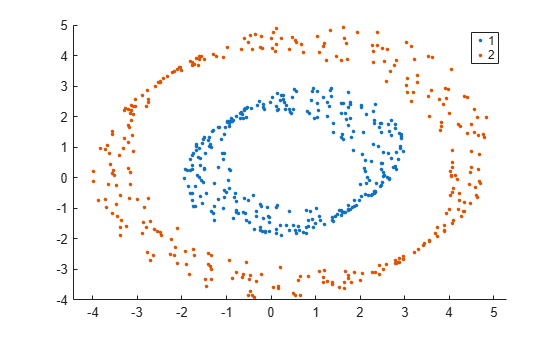The `spectralcluster` function correctly identifies the two clusters in the data set.

Compute a similarity matrix from Fisher's iris data set and perform spectral clustering on the similarity matrix.

Load Fisher's iris data set. Use the petal lengths and widths as features to consider for clustering.

```load fisheriris X = meas(:,3:4); gscatter(X(:,1),X(:,2),species);```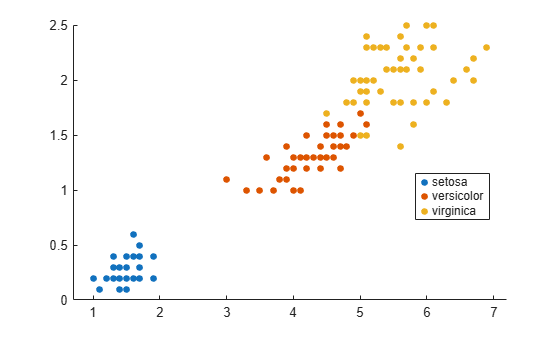Find the distance between each pair of observations in `X` by using the `pdist` and `squareform` functions with the default Euclidean distance metric.

```dist_temp = pdist(X); dist = squareform(dist_temp);```

Construct the similarity matrix and confirm that it is symmetric.

```S = exp(-dist.^2); issymmetric(S)```
```ans = logical 1 ```

Perform spectral clustering. Specify `'Distance','precomputed'` to perform clustering using the similarity matrix. Specify `k=3` clusters, and set the `'LaplacianNormalization'` name-value pair argument to use the normalized symmetric Laplacian matrix.

```k = 3; % Number of clusters rng('default') % For reproducibility idx = spectralcluster(S,k,'Distance','precomputed','LaplacianNormalization','symmetric');```

`idx` contains the cluster indices for each observation in `X`.

Visualize the result of clustering.

`gscatter(X(:,1),X(:,2),idx);`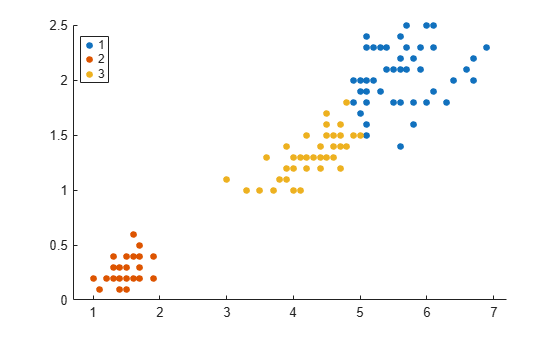Tabulate the clustering results.

`tabulate(idx)`
``` Value Count Percent 1 48 32.00% 2 50 33.33% 3 52 34.67% ```

The `Percent` column shows the percentage of data points assigned to the three clusters.

Repeat spectral clustering using the data as input to `spectralcluster`. Specify `'NumNeighbors'` as `size(X,1)`, which corresponds to creating the similarity matrix `S` by connecting each point to all the remaining points.

```idx2 = spectralcluster(X,k,'NumNeighbors',size(X,1),'LaplacianNormalization','symmetric'); gscatter(X(:,1),X(:,2),idx2);```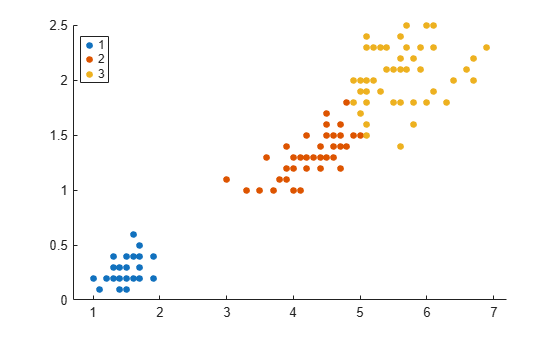`tabulate(idx2)`
``` Value Count Percent 1 50 33.33% 2 52 34.67% 3 48 32.00% ```

The clustering results for both approaches are the same. The order of cluster assignments is different, even though the data points are clustered in the same way.

Find clusters in a data set, based on a specified search radius for creating a similarity graph.

Create data with `3` clusters, each containing 500 points.

```rng('default') % For reproducibility N = 500; X = [mvnrnd([0 0],eye(2),N); ... mvnrnd(5*[1 -1],eye(2),N); ... mvnrnd(5*[1 1],eye(2),N)];```

Specify a search radius of `2` for creating a similarity graph, and find 3 clusters in the data.

`idx = spectralcluster(X,3,'SimilarityGraph','epsilon','Radius',2);`

Visualize the result of clustering.

`gscatter(X(:,1),X(:,2),idx);`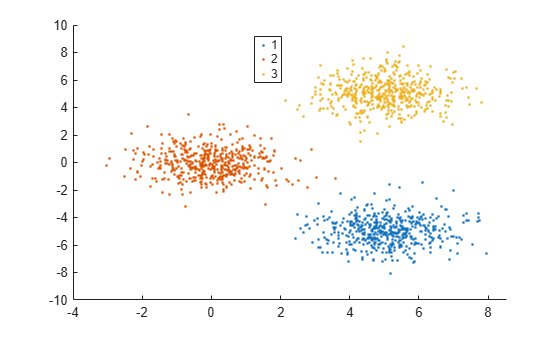Find the eigenvalues and eigenvectors of the Laplacian matrix and use the values to confirm clustering results.

Randomly generate sample data with three well-separated clusters, each containing 100 points.

```rng('default'); % For reproducibility n = 100; X = [randn(n,2)*0.5+3; randn(n,2)*0.5 randn(n,2)*0.5-3]; ```

Estimate the number of clusters in the data by using the eigenvalues of the Laplacian matrix. Compute the five smallest eigenvalues (in magnitude) of the Laplacian matrix.

`[~,~,D_temp] = spectralcluster(X,5)`
```D_temp = 5×1 -0.0000 -0.0000 -0.0000 0.0277 0.0296 ```

Only the first three eigenvalues are approximately zero. The number of zero eigenvalues is a good indicator of the number of connected components in a similarity graph and, therefore, is a good estimate of the number of clusters in your data. So, `k=3` is a good estimate of the number of clusters in `X`.

Find `k=3` clusters and return the three smallest eigenvalues and corresponding eigenvectors of the Laplacian matrix.

`[idx,V,D] = spectralcluster(X,3)`
```idx = 300×1 3 3 3 3 3 3 3 3 3 3 ⋮ ```
```V = 300×3 -0.0000 0.1000 0.0000 -0.0000 0.1000 0.0000 -0.0000 0.1000 0.0000 -0.0000 0.1000 0.0000 -0.0000 0.1000 0.0000 -0.0000 0.1000 0.0000 -0.0000 0.1000 0.0000 -0.0000 0.1000 0.0000 -0.0000 0.1000 0.0000 -0.0000 0.1000 0.0000 ⋮ ```
```D = 3×1 10-16 × -0.3634 -0.3857 -0.3894 ```

Elements of `D` correspond to the three smallest eigenvalues of the Laplacian matrix. The columns of `V` contain the eigenvectors corresponding to the eigenvalues in `D`. For well-separated clusters, the eigenvectors are indicator vectors. The eigenvectors have values of zero (or close to zero) for points that do not belong to a particular cluster, and nonzero values for points that belong to a particular cluster.

Visualize the result of clustering.

`gscatter(X(:,1),X(:,2),idx);`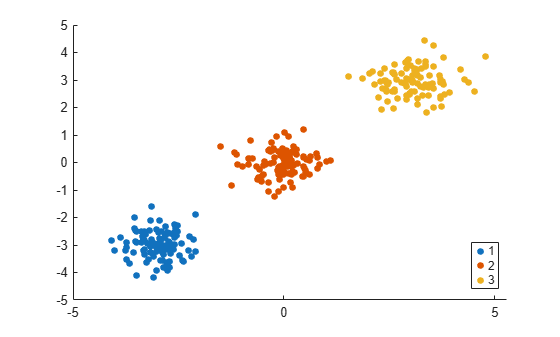## Input Arguments

collapse all

Input data, specified as an n-by-p numeric matrix. The rows of `X` correspond to observations (or points), and the columns correspond to variables.

The software treats `NaN`s in `X` as missing data and ignores any row of `X` containing at least one `NaN`. The `spectralcluster` function returns `NaN` values for the corresponding row in the output arguments `idx` and `V`.

Data Types: `single` | `double`

Similarity matrix, specified as an n-by-n symmetric matrix, where n is the number of observations. A similarity matrix (or adjacency matrix) represents the input data by modeling local neighborhood relationships among the data points. The values in a similarity matrix represent the edges (or connections) between nodes (data points) that are connected in a similarity graph. For more information, see Similarity Matrix.

`S` must not contain any `NaN` values.

To use a similarity matrix as the first input of `spectralcluster`, you must specify `'Distance','precomputed'`.

Data Types: `single` | `double`

Number of clusters in the data, specified as a positive integer.

For details about how to estimate the number of clusters, see Tips.

Data Types: `single` | `double`

### Name-Value Pair Arguments

Specify optional comma-separated pairs of `Name,Value` arguments. `Name` is the argument name and `Value` is the corresponding value. `Name` must appear inside quotes. You can specify several name and value pair arguments in any order as `Name1,Value1,...,NameN,ValueN`.

Example: `spectralcluster(X,3,'SimilarityGraph','epsilon','Radius',5)` specifies `3` clusters and uses the radius search method with a search radius of `5` to construct a similarity graph.

Distance metric, specified as the comma-separated pair consisting of `'Distance'` and a character vector, string scalar, or function handle, as described in this table.

ValueDescription
`'precomputed'`

Precomputed distance. You must specify this option if the first input to `spectralcluster` is a similarity matrix `S`.

`'euclidean'`

Euclidean distance (default)

`'seuclidean'`

Standardized Euclidean distance. Each coordinate difference between observations is scaled by dividing by the corresponding element of the standard deviation computed from `X`. Use the `Scale` name-value pair argument to specify a different scaling factor.

`'mahalanobis'`

Mahalanobis distance using the sample covariance of `X`, `C = nancov(X)`. Use the `Cov` name-value pair argument to specify a different covariance matrix.

`'cityblock'`

City block distance

`'minkowski'`

Minkowski distance. The default exponent is 2. Use the `P` name-value pair argument to specify a different exponent, where `P` is a positive scalar value.

`'chebychev'`

Chebychev distance (maximum coordinate difference)

`'cosine'`

One minus the cosine of the included angle between observations (treated as vectors)

`'correlation'`

One minus the sample correlation between observations (treated as sequences of values)

`'hamming'`

Hamming distance, which is the percentage of coordinates that differ

`'jaccard'`

One minus the Jaccard coefficient, which is the percentage of nonzero coordinates that differ

`'spearman'`

One minus the sample Spearman's rank correlation between observations (treated as sequences of values)

`@distfun`

Custom distance function handle. A distance function has the form

```function D2 = distfun(ZI,ZJ) % calculation of distance ...```
where

• `ZI` is a `1`-by-`n` vector containing a single observation.

• `ZJ` is an `m2`-by-`n` matrix containing multiple observations. `distfun` must accept a matrix `ZJ` with an arbitrary number of observations.

• `D2` is an `m2`-by-`1` vector of distances, and `D2(k)` is the distance between observations `ZI` and `ZJ(k,:)`.

If your data is not sparse, you can generally compute distance more quickly by using a built-in distance instead of a function handle.

When you use the `'seuclidean'`, `'minkowski'`, or `'mahalanobis'` distance metric, you can specify the additional name-value pair argument `'Scale'`, `'P'`, or `'Cov'`, respectively, to control the distance metric.

Example: `spectralcluster(X,5,'Distance','minkowski','P',3)` specifies `5` clusters and uses of the Minkowski distance metric with an exponent of `3` to perform the clustering algorithm.

Exponent for the Minkowski distance metric, specified as the comma-separated pair consisting of `'P'` and a positive scalar.

This argument is valid only if `'Distance'` is `'minkowski'`.

Example: `'P',3`

Data Types: `single` | `double`

Covariance matrix for the Mahalanobis distance metric, specified as the comma-separated pair consisting of `'Cov'` and a positive definite matrix.

This argument is valid only if `'Distance'` is `'mahalanobis'`.

Example: `'Cov',eye(4)`

Data Types: `single` | `double`

Scaling factors for the standardized Euclidean distance metric, specified as the comma-separated pair consisting of `'Scale'` and a numeric vector of nonnegative values.

`Scale` has length p (the number of columns in `X`), because each dimension (column) of `X` has a corresponding value in `Scale`. For each dimension of `X`, `spectralcluster` uses the corresponding value in `Scale` to standardize the difference between observations.

This argument is valid only if `'Distance'` is `'seuclidean'`.

Data Types: `single` | `double`

Type of similarity graph to construct from the input data `X`, specified as the comma-separated pair consisting of `'SimilarityGraph'` and one of these values.

ValueDescriptionGraph-Specific Name-Value Pair Arguments
`'knn'`(Default) Construct the graph using nearest neighbors.

`'NumNeighbors'` — Number of nearest neighbors used to construct the similarity graph

`'KNNGraphType'` — Type of nearest neighbor graph

`'epsilon'`

Construct the graph using a radius search. You must specify a value for `Radius` if you use this option.

`'Radius'` — Search radius for the nearest neighbors used to construct the similarity graph

This argument is valid only if `'Distance'` is not `'precomputed'`.

Example: `'SimilarityGraph','epsilon'`

Number of nearest neighbors used to construct the similarity graph, specified as the comma-separated pair consisting of `'NumNeighbors'` and a positive integer.

This argument is valid only if `'SimilarityGraph'` is `'knn'`. For more information, see Similarity Graph.

Example: `'NumNeighbors',10`

Data Types: `single` | `double`

Type of nearest neighbor graph, specified as the comma-separated pair consisting of `'KNNGraphType'` and one of these values.

ValueDescription
`'complete'`

(Default) Connects two points i and j, when either i is a nearest neighbor of j or j is a nearest neighbor of i.

This option leads to a denser representation of the similarity matrix.

`'mutual'`

Connects two points i and j, when i is a nearest neighbor of j and j is a nearest neighbor of i.

This option leads to a sparser representation of the similarity matrix.

This argument is valid only if `'SimilarityGraph'` is `'knn'`.

Example: `'KNNGraphType','mutual'`

Search radius for the nearest neighbors used to construct the similarity graph, specified as the comma-separated pair consisting of `'Radius'` and a nonnegative scalar.

You must specify this argument if `'SimilarityGraph'` is `'epsilon'`. For more information, see Similarity Graph.

Example: `'Radius',5`

Data Types: `single` | `double`

Scale factor for the kernel, specified as the comma-separated pair consisting of `'KernelScale'` and `'auto'` or a positive scalar. The software uses the scale factor to transform distances to similarity measures. For more information, see Similarity Graph.

• The `'auto'` option is supported only for the `'euclidean'` and `'seuclidean'` distance metrics.

• If you specify `'auto'`, then the software selects an appropriate scale factor using a heuristic procedure. This heuristic procedure uses subsampling, so estimates can vary from one call to another. To reproduce results, set a random number seed using `rng` before calling `spectralcluster`.

This argument is valid only if `'Distance'` is not `'precomputed'`.

Example: `'KernelScale','auto'`

Data Types: `double` | `single` | `char` | `string`

Method to normalize the Laplacian matrix L, specified as the comma-separated pair consisting of `'LaplacianNormalization'` and one of these values.

ValueDescription
`'none'`

Use Laplacian matrix L without normalization.

`'randomwalk'`

(Default) Use the normalized random-walk Laplacian matrix Lrw (Shi-Malik ).

`${L}_{rw}={D}_{g}{}^{-1}L.$`

The matrix Dg is the degree matrix.

`'symmetric'`

Use the normalized symmetric Laplacian matrix Ls (Ng-Jordan-Weiss ).

`${L}_{s}={D}_{g}{}^{-1/2}L{D}_{g}{}^{-1/2}.$`

The matrix Dg is the degree matrix.

Example: `'LaplacianNormalization','randomwalk'`

Clustering method to cluster the eigenvectors of the Laplacian matrix, specified as the comma-separated pair consisting of `'ClusterMethod'` and either `'kmeans'` or `'kmedoids'`.

`kmeans` and `kmedoids` involve randomness in their algorithms. Therefore, to reproduce the results of `spectralcluster`, you must set the seed of the random number generator by using `rng`.

Example: `'ClusterMethod','kmedoids'`

## Output Arguments

collapse all

Cluster indices, returned as a numeric column vector. `idx` has n rows, and each row of `idx` indicates the cluster assignment of the corresponding row (or observation) in `X`.

Data Types: `double`

Eigenvectors, returned as an n-by-`k` numeric matrix. The columns of `V` are the eigenvectors corresponding to the `k` smallest eigenvalues of the Laplacian matrix. These eigenvectors are a low-dimensional representation of the input data `X` in a new space where clusters are more widely separated.

For well-separated clusters, the eigenvectors are indicator vectors. That is, the eigenvectors have values of zero (or close to zero) for points that do not belong to a given cluster, and nonzero values for points that belong to a particular cluster.

Data Types: `single` | `double`

Eigenvalues, returned as a `k`-by-1 numeric vector that contains the `k` smallest eigenvalues of the Laplacian matrix. The number of zero eigenvalues in `D` is an indicator of the number of connected components in the similarity graph and, therefore, is a good estimate of the number of clusters in your data.

Data Types: `single` | `double`

collapse all

### Similarity Graph

A similarity graph models the local neighborhood relationships between data points in `X` as an undirected graph. The nodes in the graph represent data points, and the edges, which are directionless, represent the connections between the data points.

If the pairwise distance Disti,j between any two nodes i and j is positive (or larger than a certain threshold), then the similarity graph connects the two nodes using an edge . The edge between the two nodes is weighted by the pairwise similarity Si,j, where ${S}_{i,j}=\mathrm{exp}\left(-{\left(\frac{Dis{t}_{i,j}}{\sigma }\right)}^{2}\right)$, for a specified kernel scale σ value.

`spectralcluster` supports these two methods of constructing a similarity graph:

• Nearest neighbor method (if `'SimilarityGraph'` is `'knn'`(default)): `spectralcluster` connects points in `X` that are nearest neighbors. You can use the `'NumNeighbors'` and `'KNNGraphType'` name-value pair arguments to specify the options for constructing the nearest neighbor graph.

• Use `'NumNeighbors'` to specify the number of nearest neighbors.

• Use `'KNNGraphType'` to specify whether to make a `'complete'` or `'mutual'` connection of points.

• Radius search method (if `'SimilarityGraph'` is `'epsilon'`): `spectralcluster` connects points whose pairwise distances are smaller than a search radius. You must specify the search radius for nearest neighbors used to construct the similarity graph by using the `'Radius'` name-value pair argument.

### Similarity Matrix

A similarity matrix is a matrix representation of a similarity graph. The n-by-n matrix $S={\left({S}_{i,j}\right)}_{i,j=1,\text{\hspace{0.17em}}\dots ,\text{\hspace{0.17em}}n}$ contains pairwise similarity values between connected nodes in the similarity graph. The similarity matrix of a graph is also called an adjacency matrix.

The similarity matrix is symmetric because the edges of the similarity graph are directionless. A value of ```Si,j = 0``` means that nodes i and j of the similarity graph are not connected.

### Degree Matrix

A degree matrix Dg is an n-by-n diagonal matrix obtained by summing the rows of the similarity matrix S. That is, the ith diagonal element of Dg is ${D}_{g}\left(i,i\right)=\sum _{j=1}^{n}{S}_{i,j}.$

### Laplacian Matrix

A Laplacian matrix is one way of representing a similarity graph. The `spectralcluster` function supports the unnormalized Laplacian matrix, the normalized Laplacian matrix using the Shi-Malik method , and the normalized Laplacian matrix using the Ng-Jordan-Weiss method .

• The unnormalized Laplacian matrix L is the difference between the degree matrix and the similarity matrix.

`$L={D}_{g}-S.$`

• The normalized random-walk Laplacian matrix (Shi-Malik) is defined as:

`${L}_{rw}={D}_{g}{}^{-1}L.$`

To derive Lrw, solve the generalized eigenvalue problem $Lv=\lambda {D}_{g}v$, where v is a column vector of length n, and λ is a scalar. The values of λ that satisfy the equation are the generalized eigenvalues of the matrix ${L}_{rw}={D}_{g}{}^{-1}L.$

You can use the MATLAB® function `eigs` to solve the generalized eigenvalue problem.

• The normalized symmetric Laplacian matrix (Ng-Jordan-Weiss) is defined as:

`${L}_{s}={D}_{g}{}^{-1/2}L{D}_{g}{}^{-1/2}.$`

Use the `'LaplacianNormalization'` name-value pair argument to specify the method to normalize the Laplacian matrix.

## Tips

• Consider using spectral clustering when the clusters in your data do not naturally correspond to convex regions.

• From the spectral clustering algorithm, you can estimate the number of clusters `k` as:

• The number of eigenvalues of the Laplacian matrix that are equal to `0`.

• The number of connected components in your similarity graph representation. Use `graph` to create a similarity graph from a similarity matrix, and use `conncomp` to find the number of connected components in the graph.

For an example, see Estimate Number of Clusters and Perform Spectral Clustering.

## Algorithms

Spectral clustering is a graph-based algorithm for clustering data points (or observations in `X`). The algorithm involves constructing a graph, finding its Laplacian matrix, and using this matrix to find k eigenvectors to split the graph k ways. By default, the algorithm for `spectralcluster` computes the normalized random-walk Laplacian matrix using the method described by Shi-Malik . `spectralcluster` also supports the unnormalized Laplacian matrix and the normalized symmetric Laplacian matrix which uses the Ng-Jordan-Weiss method . `spectralcluster` implements clustering as follows:

1. For each data point in `X`, define a local neighborhood using either the radius search method or nearest neighbor method, as specified by the `'SimilarityGraph'` name-value pair argument (see Similarity Graph). Then, find the pairwise distances $Dis{t}_{i,j}$ for all points i and j in the neighborhood.

2. Convert the distances to similarity measures using the kernel transformation ${S}_{i,j}=\mathrm{exp}\left(-{\left(\frac{Dis{t}_{i,j}}{\sigma }\right)}^{2}\right)$. The matrix S is the similarity matrix, and σ is the scale factor for the kernel, as specified using the `'KernelScale'` name-value pair argument.

3. Calculate the unnormalized Laplacian matrix L , the normalized random-walk Laplacian matrix Lrw, or the normalized symmetric Laplacian matrix Ls, depending on the value of the `'LaplacianNormalization'` name-value pair argument.

4. Create a matrix $V\in {ℝ}^{n×k}$ containing columns ${v}_{1},\dots ,{v}_{k}$, where the columns are the k eigenvectors that correspond to the k smallest eigenvalues of the Laplacian matrix. If using Ls, normalize each row of V to have unit length.

5. Treating each row of V as a point, cluster the n points using k-means clustering (default) or k-medoids clustering, as specified by the `'ClusterMethod'` name-value pair argument.

6. Assign the original points in `X` to the same clusters as their corresponding rows in V.

 Von Luxburg, U. “A Tutorial on Spectral Clustering.” Statistics and Computing Journal. Vol.17, Number 4, 2007, pp. 395–416.

 Shi, J., and J. Malik. “Normalized cuts and image segmentation.” IEEE Transactions on Pattern Analysis and Machine Intelligence. Vol. 22, 2000, pp. 888–905.

 Ng, A.Y., M. Jordan, and Y. Weiss. “On spectral clustering: Analysis and an algorithm.” In Proceedings of the Advances in Neural Information Processing Systems 14. MIT Press, 2001, pp. 849–856.